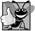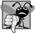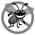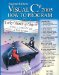# Examples Using Arrays

This section presents several examples that demonstrate declaring arrays, creating arrays, initializing arrays and manipulating array elements.

Creating and Initializing an Array

The application of Fig. 8.2 uses keyword new to create an array of 10 int elements that are initially 0 (the default for int variables).

Figure 8.2. Creating an array.

``` 1 // Fig. 8.2: InitArray.cs
2 // Creating an array.
3 using System;
4
5 public class InitArray
6 {
7 public static void Main( string[] args )
8 {
9 int[] array; // declare array named array
10
11 // create the space for array and initialize to default zeros
12 array = new int[ 10 ]; // 10 int elements
13
14 Console.WriteLine( "{0}{1,8}", "Index", "Value" ); // headings
15
16 // output each array element's value
17 for ( int counter = 0; counter < array.Length; counter++ )
18  Console.WriteLine( "{0,5}{1,8}", counter, array[ counter ] );
19 } // end Main
20 } // end class InitArray
```

 ``` Index Value 0 0 1 0 2 0 3 0 4 0 5 0 6 0 7 0 8 0 9 0 ```

Line 9 declares arraya reference capable of referring to an array of int elements. Line 12 creates the 10-element array object and assigns its reference to variable array. Line 14 outputs the column headings. The first column contains the index (09) of each array element, and the second column contains the default value (0) of each array element and has a field width of 8.

The for statement in lines 1718 outputs the index number (represented by counter) and the value (represented by array[ counter ]) of each array element. Note that the loop control variable counter is initially 0index values start at 0, so using zero-based counting allows the loop to access every element of the array. The for statement's loop-continuation condition uses the property array.Length (line 17) to obtain the length of the array. In this example, the length of the array is 10, so the loop continues executing as long as the value of control variable counter is less than 10. The highest index value of a 10-element array is 9, so using the less-than operator in the loop-continuation condition guarantees that the loop does not attempt to access an element beyond the end of the array (i.e., during the final iteration of the loop, counter is 9). We will soon see what happens when such an out-of-range index is encountered at execution time.

Using an Array Initializer

An application can create an array and initialize its elements with an array initializer, which is a comma-separated list of expressions (called an initializer list) enclosed in braces. In this case, the array length is determined by the number of elements in the initializer list. For example, the declaration

```int[] n = { 10, 20, 30, 40, 50 };
```

creates a five-element array with index values 0, 1, 2, 3 and 4. Element n[ 0 ] is initialized to 10, n[ 1 ] is initialized to 20 and so on. This declaration does not require new to create the array object. When the compiler encounters an array declaration that includes an initializer list, the compiler counts the number of initializers in the list to determine the size of the array, then sets up the appropriate new operation "behind the scenes."

The application in Fig. 8.3 initializes an integer array with 10 values (line 10) and displays the array in tabular format. The code for displaying the array elements (lines 1516) is identical to that in Fig. 8.2 (lines 1718).

Figure 8.3. Initializing the elements of an array with an array initializer.

``` 1 // Fig. 8.3: InitArray.cs
2 // Initializing the elements of an array with an array initializer.
3 using System;
4
5 public class InitArray
6 {
7 public static void Main( string[] args )
8 {
9 // initializer list specifies the value for each element
10 int[] array = { 32, 27, 64, 18, 95, 14, 90, 70, 60, 37 };
11
12 Console.WriteLine( "{0}{1,8}", "Index", "Value" ); // headings
13
14 // output each array element's value
15 for ( int counter = 0; counter < array.Length; counter++ )
16 Console.WriteLine( "{0,5}{1,8}", counter, array[ counter ] );
17 } // end Main
18 } // end class InitArray
```

 ``` Index Value 0 32 1 27 2 64 3 18 4 95 5 14 6 90 7 70 8 60 9 37 ```

Calculating a Value to Store in Each Array Element

Some applications calculate the value to be stored in each array element. The application in Fig. 8.4 creates a 10-element array and assigns to each element one of the even integers from 2 to 20 (2, 4, 6, ..., 20). Then the application displays the array in tabular format. The for statement at lines 1314 calculates an array element's value by multiplying the current value of the for loop's control variable counter by 2, then adding 2.

Figure 8.4. Calculating values to be placed into the elements of an array.

``` 1 // Fig. 8.4: InitArray.cs
2 // Calculating values to be placed into the elements of an array.
3 using System;
4
5 public class InitArray
6 {
7 public static void Main( string[] args )
8 {
9 const int ARRAY_LENGTH = 10; // create a named constant
10 int[] array = new int[ ARRAY_LENGTH ]; // create array
11
12 // calculate value for each array element
13 for ( int counter = 0; counter < array.Length; counter++ )
14 array[ counter ] = 2 + 2 * counter;
15
```
16 Console.WriteLine( "{0}{1,8}", "Index", "Value" ); // headings 17 18 // output each array element's value 19 for ( int counter = 0; counter < array.Length; counter++ ) 20 Console.WriteLine( "{0,5}{1,8}", counter, array[ counter ] ); 21 } // end Main 22 } // end class InitArray
 ``` Index Value 0 2 1 4 2 6 3 8 4 10 5 12 6 14 7 16 8 18 9 20 ```

Line 9 uses the modifier const to declare the constant ARRAY_LENGTH, whose value is 10. Constants must be initialized when they are declared and cannot be modified thereafter. Note that constants, like the enum constants introduced in Section 7.10, are declared with all capital letters by convention, to make them stand out in the code.## Good Programming Practice 8 2

Constants also are called named constants. Such variables often make applications more readable than applications that use literal values (e.g., 10)a named constant such as ARRAY_LENGTH clearly indicates its purpose, whereas a literal value could have different meanings based on the context in which it is used. Another advantage to using named constants is that if the value of the constant must be changed, it is necessary to change it only in the declaration, thus reducing the cost of maintaining the code.## Common Programming Error 8 2

Assigning a value to a named constant after it has been initialized is a compilation error.## Common Programming Error 8 3

Attempting to declare a named constant without initializing it is a compilation error.

Summing the Elements of an Array

Often, the elements of an array represent a series of values to be used in a calculation. For example, if the elements of an array represent exam grades, an instructor may wish to total the elements of the array and use that total to calculate the class average for the exam. The GradeBook examples later in the chapter (Fig. 8.15 and Fig. 8.20) use this technique.

The application in Fig. 8.5 sums the values contained in a 10-element integer array. The application declares, creates and initializes the array at line 9. The for statement performs the calculations. [Note: The values supplied as array initializers are often read into an application, rather than specified in an initializer list. For example, an application could input the values from a user or from a file on disk (as discussed in Chapter 18, Files and Streams). Reading the data into an application makes the application more reusable, because it can be used with different sets of data.]

Figure 8.5. Computing the sum of the elements of an array.

(This item is displayed on page 343 in the print version)

``` 1 // Fig. 8.5: SumArray.cs
2 // Computing the sum of the elements of an array.
3 using System;
4
5 public class SumArray
6 {
7 public static void Main( string[] args )
8 {
9 int[] array = { 87, 68, 94, 100, 83, 78, 85, 91, 76, 87 };
10 int total = 0;
11
12 // add each element's value to total
13 for ( int counter = 0; counter < array.Length; counter++ )
14  total += array[ counter ];
15
16 Console.WriteLine( "Total of array elements: {0}", total );
17 } // end Main
18 } // end class SumArray
```

 ``` Total of array elements: 849 ```

Using Bar Charts to Display Array Data Graphically

Many applications present data to users in a graphical manner. For example, numeric values are often displayed as bars in a bar chart. In such a chart, longer bars represent proportionally larger numeric values. One simple way to display numeric data graphically is with a bar chart that shows each numeric value as a bar of asterisks (*).

Figure 8.6. Bar chart printing application.

(This item is displayed on page 344 in the print version)

``` 1 // Fig. 8.6: BarChart.cs
2 // Bar chart printing application.
3 using System;
4
5 public class BarChart
6 {
7 public static void Main( string[] args )
8 {
9 int[] array = { 0, 0, 0, 0, 0, 0, 1, 2, 4, 2, 1 };
10
12
13 // for each array element, output a bar of the chart
14 for ( int counter = 0; counter < array.Length; counter++ )
15 {
16 // output bar labels ( "00-09: ", ..., "90-99: ", "100: " )
17 if ( counter == 10 )
18 Console.Write( " 100: " );
19 else
20 Console.Write( "{0:D2}-{1:D2}: ",
21 counter * 10, counter * 10 + 9 );
22
23 // print bar of asterisks
24 for ( int stars = 0; stars < array[ counter ]; stars++ )
25  Console.Write( "*" );
26
27 Console.WriteLine(); // start a new line of output
28 } // end outer for
29 } // end Main
30 } // end class BarChart
```

 ``` Grade distribution: 00-09: 10-19: 20-29: 30-39: 40-49: 50-59: 60-69: * 70-79: ** 80-89: **** 90-99: ** 100: * ```

The application reads the numbers from the array and graphs the information as a bar chart. Each grade range is followed by a bar of asterisks indicating the number of grades in that range. To label each bar, lines 1721 output a grade range (e.g., "70-79: ") based on the current value of counter. When counter is 10, line 18 outputs " 100: " to align the colon with the other bar labels. When counter is not 10, line 20 uses the format items {0:D2} and {1:D2} to output the label of the grade range. The format specifier D indicates that the value should be formatted as an integer, and the number after the D indicates how many digits this formatted integer must contain. The 2 indicates that values with fewer than two digits should begin with a leading 0.

The nested for statement (lines 2425) outputs the bars. Note the loop-continuation condition at line 24 (stars < array[ counter ]). Each time the application reaches the inner for, the loop counts from 0 up to one less than array[ counter ], thus using a value in array to determine the number of asterisks to display. In this example, array[ 0 ]array[ 5 ] contain 0s because no students received a grade below 60. Thus, the application displays no asterisks next to the first six grade ranges.

Using the Elements of an Array as Counters

Sometimes, applications use counter variables to summarize data, such as the results of a survey. In Fig. 7.8, we used separate counters in our die-rolling application to track the number of times each face of a six-sided die appeared as the application rolled the die 6000 times. An array version of the application in Fig. 7.8 is shown in Fig. 8.7.

Figure 8.7. Roll a six-sided die 6000 times.

``` 1 // Fig. 8.7: RollDie.cs
2 // Roll a six-sided die 6000 times.
3 using System;
4
5 public class RollDie
6 {
7 public static void Main( string[] args )
8 {
9 Random randomNumbers = new Random(); // random number generator
10 int[] frequency = new int[ 7 ]; // array of frequency counters
11
12 // roll die 6000 times; use die value as frequency index
13 for ( int roll = 1; roll <= 6000; roll++ )
14 ++frequency[ randomNumbers.Next( 1, 7 ) ];
15
16 Console.WriteLine( "{0}{1,10}", "Face", "Frequency" );
17
18 // output each array element's value
19 for ( int face = 1; face < frequency.Length; face++ )
20 Console.WriteLine( "{0,4}{1,10}", face, frequency[ face ] );
21 } // end Main
22 } // end class RollDie
```

 ``` Face Frequency 1 956 2 981 3 1001 4 1030 5 1035 6 997 ```

Fig. 8.7 uses array frequency (line 10) to count the occurrences of each side of the die. The single statement in line 14 of this application replaces lines 2346 of Fig. 7.8. Line 14 uses the random value to determine which frequency element to increment during each iteration of the loop. The calculation in line 14 produces random numbers from 1 to 6, so array frequency must be large enough to store six counters. We use a seven-element array in which we ignore frequency[ 0 ]it is more logical to have the face value 1 increment frequency[ 1 ] than frequency[ 0 ]. Thus, each face value is used as an index for array frequency. We also replaced lines 5052 of Fig. 7.8 by looping through array frequency to output the results (Fig. 8.7, lines 1920).

Using Arrays to Analyze Survey Results

Our next example uses arrays to summarize the results of data collected in a survey:

Forty students were asked to rate the quality of the food in the student cafeteria on a scale of 1 to 10 (where 1 means awful and 10 means excellent). Place the 40 responses in an integer array and summarize the results of the poll.

This is a typical array-processing application (see Fig. 8.8). We wish to summarize the number of responses of each type (i.e., 1 through 10). The array responses (lines 1012) is a 40-element int array of the students' responses to the survey. We use 11-element array frequency (line 13) to count the number of occurrences of each response. Each element of the array is used as a counter for one of the survey responses and is initialized to 0 by default. As in Fig. 8.7, we ignore frequency[ 0 ].

Figure 8.8. Poll analysis application.

``` 1 // Fig. 8.8: StudentPoll.cs
2 // Poll analysis application.
3 using System;
4
5 public class StudentPoll
6 {
7 public static void Main( string[] args )
8 {
9 // array of survey responses
10 int[] responses = { 1, 2, 6, 4, 8, 5, 9, 7, 8, 10, 1, 6, 3, 8, 6,
11 10, 3, 8, 2, 7, 6, 5, 7, 6, 8, 6, 7, 5, 6, 6, 5, 6, 7, 5, 6,
12 4, 8, 6, 8, 10 };
13 int[] frequency = new int[ 11 ]; // array of frequency counters
14
15 // for each answer, select responses element and use that value
16 // as frequency index to determine element to increment
18 ++frequency[ responses[ answer ] ];
19
20 Console.WriteLine( "{0}{1,10}", "Rating", "Frequency" );
21
22 // output each array element's value
23 for ( int rating = 1; rating < frequency.Length; rating++ )
24 Console.WriteLine( "{0,6}{1,10}", rating, frequency[ rating ] );
25 } // end Main
26 } // end class StudentPoll
```

 ``` Rating Frequency 1 2 2 2 3 2 4 2 5 5 6 11 7 5 8 7 9 1 10 3 ```

The for loop at lines 1718 takes the responses one at a time from array responses and increments one of the 10 counters in the frequency array (frequency[ 1 ] to frequency[ 10 ]). The key statement in the loop is line 18, which increments the appropriate frequency counter, depending on the value of responses[ answer ].

Let's consider several iterations of the for loop. When control variable answer is 0, the value of responses[ answer ] is the value of responses[ 0 ] (i.e., 1 in line 10), so the application interprets ++frequency[ responses[ answer ] ] as

```++frequency[ 1 ]
```

which increments the value in frequency array element 1. To evaluate the expression, start with the value in the innermost set of square brackets, answer. Once you know answer's value (which is the value of the loop control variable in line 17), plug it into the expression and evaluate the next outer set of square bracketsi.e., responses[ answer ], which is a value selected from the responses array in lines 1012. Then use the resulting value as the index for the frequency array to specify which counter to increment (line 18).

When answer is 1, responses[ answer ] is the value of responses[ 1 ], which is 2, so the application interprets ++frequency[ responses[ answer ] ] as

```++frequency[ 2 ]
```

which increments the frequency array element 2.

When answer is 2, responses[ answer ] is the value of responses[ 2 ], which is 6, so the application interprets ++frequency[ responses[ answer ] ] as

```++frequency[ 6 ]
```

which increments frequency array element 6, and so on. Regardless of the number of responses processed in the survey, the application requires only an 11-element array (in which we ignore element 0) to summarize the results, because all the response values are between 1 and 10, inclusive, and the index values for an 11-element array are 0 through 10.

If the data in the responses array had contained invalid values, such as 13, the application would have attempted to add 1 to frequency[ 13 ], which is outside the bounds of the array. In many programming languages, like C and C++, writing outside the bounds of an array is actually allowed and would overwrite arbitrary information in memory, often causing disastrous results. C# does not allow thisaccessing any array element forces a check on the array index to ensure that it is valid (i.e., it must be greater than or equal to 0 and less than the length of the array). This is called bounds checking. If an application uses an invalid index, the Common Language Runtime generates an exception (specifically, an IndexOutOfRangeException) to indicate that an error occurred in the application at execution time. The condition in a control statement could determine whether an index is valid before allowing it to be used in an array-access expression, thus avoiding the exception.## Error Prevention Tip 8 1

An exception indicates that an error has occurred in an application. You often can write code to recover from an exception and continue application execution, rather than abnormally terminating the application. Exception handling is discussed in Chapter 12.## Error Prevention Tip 8 2

When writing code to loop through an array, ensure that the array index remains greater than or equal to 0 and less than the length of the array. The loop-continuation condition should prevent the accessing of elements outside this range.

### Case Study Card Shuffling and Dealing SimulationVisual C# 2005 How to Program (2nd Edition)
ISBN: 0131525239
EAN: 2147483647
Year: 2004
Pages: 600